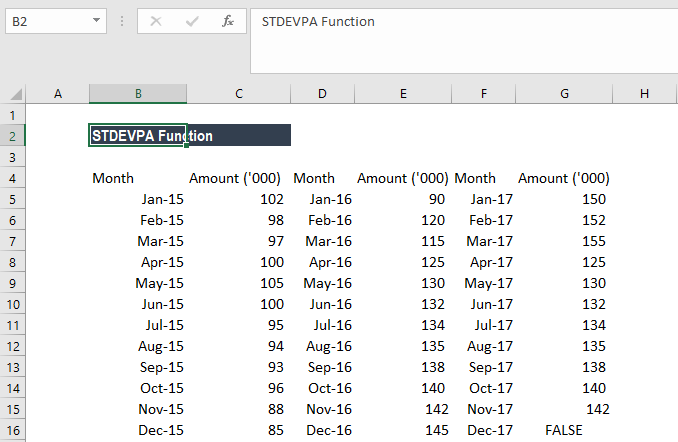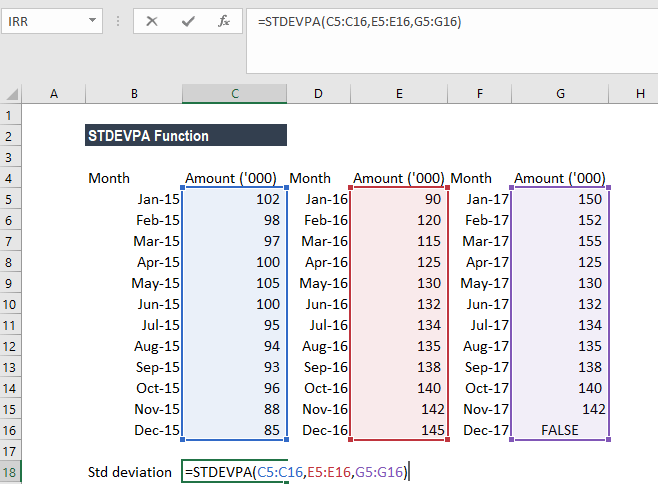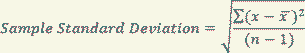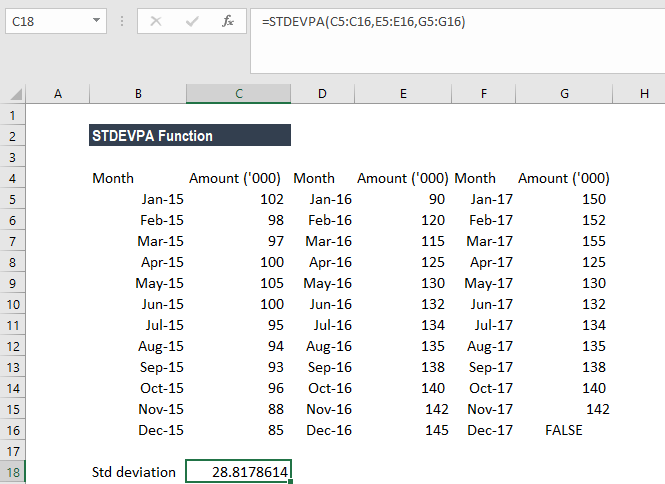# STDEVPA Function

Calculate standard deviation that is based on entire population given as arguments

## What is the STDEVPA Function?

The STDEVPA Function is categorized under Excel Statistical functions. It will calculate the standard deviation that is based on the entire population given as arguments. The function will ignore logical values and text.

As a financial analyst, the STDEVPA function can be used, for example, to calculate deviations in revenue. Portfolio managers often use standard deviation to measure and keep track of their portfolio’s risk level.

### Formula

=STDEVPA(value1, value2,…)

The STDEVPA function uses the following arguments:

1. Value1 (required argument) – This is the first number argument corresponding to a population.
2. Value2 (optional argument) – This is a number argument that corresponds to the population.

#### A few notes on the arguments

1. The given arguments must provide at least two numeric values to the function.
2. The arguments should be between 1 and 255 numeric values, arrays of values, or references to cells containing numbers. MS Excel 2007 or later versions allow us to enter up to 255 number arguments to the function. However, in Excel 2003, the function can only accept up to 30 number arguments.
3. Arguments can either be numbers or names, arrays, or references that contain numbers.
4. The STDEVPA function is used when we want to calculate the standard deviation for an entire population. If we are calculating the standard deviation of a sample, we need to use the STDEVA function or the STDEV function.

### How to use the STDEVPA Function in Excel?

To understand the uses of the STDEVPA function, let’s look at an example:

#### Example 1

Suppose we are given a record of the monthly sales figures of a business over the last three years, as shown below:For standard deviation, the formula used is:The equation used is:Where:

• takes on each value in the set
• is the average (statistical mean) of the set of values
• is the number of values

We get the result below:Remember here, the STDEVPA function will count logical values while calculating the standard deviation. The STDEVP function, however, ignores them.

### Things to remember about the STDEVPA Function

1. For large sample sizes, STDEVA and STDEVPA return approximately equal values.
2. If we do not want to include logical values and text representations of numbers in a reference as part of the calculation, use the STDEVP function.

Thanks for reading CFI’s guide to the Excel STDEVPA function. By taking the time to learn and master these functions, you’ll significantly speed up your financial analysis. To learn more, check out these additional CFI resources:

• Excel Functions for Finance